## Performing Metallurgical Calculations on Computerized Spreadsheets

### Alex Doll, P.Eng

http://www.agdconsulting.ca

## Abstract

Computerized spreadsheets are a commonly used tool in the engineering industry. Unfortunately, they are a very generic tool, and do require care when used to perform process engineering calculations. This paper describes many tools and tricks developed in the consulting engineering industry to make calculations more readable and to perform quality assurance.

## Introduction

Spreadsheets are a class of computer program that is used to perform a large number of simple calculations. Spreadsheets were one of the first classes of computer programs developed to operate on personal computers, and they are standard equipment in the office suites offered by the major PC software companies.

This paper describes a number of concepts that will make process engineering calculations performed on spreadsheets more readable and less prone to error. It is assumed that the reader is familiar with spreadsheets and is already able to create moderately complex calculations. It is also assumed that the reader is familiar with process engineering concepts like material balances and unit operations.

The spreadsheet formulas and notations used throughout this paper are based on the Lotus 1-2-3 spreadsheet program. Most other spreadsheet packages will have similar functions and syntax. The reader is encouraged to consult the documentation for his particular software to determine the exact formula and syntax to use.

The layout and organization of a spreadsheet based calculation has a huge effect on its overall usefulness. Many people build spreadsheets with little care or attention to layouts and diagrams under the mistaken assumption that the layout does not affect the underlying numbers and formulas. Sadly, the humans using these calculations are simple-minded creatures and seemingly irrelevant things like layouts and diagrams do make a large difference to the comprehension of what is being communicated.

A calculation, computerized or otherwise, is really a piece of communication between two or more people. Many engineers are unaware that their scribbles and spreadsheets often need to be checked in the process of designing a facility, or that these documents may end up as evidence in court if something goes wrong. This is the key reason why readability and transparency of methods used in a calculation are absolutely critical to the success of a calculation in effectively communicating to other engineers the true intentions and conclusions of the author.

Laying out the spreadsheet in a simple, step by step fashion will make obvious what was intended and often assists determining when something is wrong. Printouts of spreadsheet calculations must also be laid out so that another reasonably capable engineer can understand the methods of the calculation and perform manual checks without having the digital spreadsheet file. Spreadsheet calcs should contain all the elements of hand calcs including, where reasonable, the gradual reduction of a complex expression into a simpler one.

Spreadsheets that are laid out poorly can obscure small errors. For example, if a cell contains three operations nested together, then all the user will see on the print-out is a single value.

@SUM(0.15*@AVG(A2..A5),0.15*@AVG(B2..B5))

In this example, the 0.15 represents an interest rate; but because the rate is hidden inside the formula, it is not possible for the users to externally confirm what rate was used.

Reorganizing the example above into a more readable form would like something like this: divide the formula over 4 cells. The first cell, labelled "AvgA" would contain the single formula @AVG(A2..A5); the second cell, labelled "AvgB" would contain @AVG(B2..B5); the third cell, labeled "IntRate" would contain just the number "0.15"; and the fourth cell would perform the final calculation @SUM(IntRate*AvgA,IntRate*AvgB). By breaking up a complex formula into several simpler ones, it becomes easy for the user to understand the flow of the calculation and to understand when what he is looking at contains an error.

All the normal items that one would put on a hand calc should be included on computerized calcs. These include:

• Name of the calc (and calc number, if applicable)
• Name of the person performing the calc
• Date and revision information
Spreadsheet calcs should always include the filename and directory path in the printout.

#### Manually set cells

One key thing that another engineer will need to know when viewing a spreadsheet based calc is which values are calculated and which were fixed by the author. This is best accomplished by giving input cells a different appearance to calculated cells.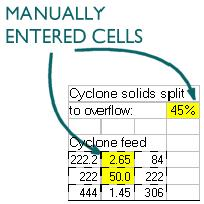The recommended way of denoting fixed cells is to give them a yellow background so that they stand out from the rest of the calc. If the output of the calc is to be used in a way that the yellow background will not appear clearly (for example, if the calc results are to be faxed), then either a font style change (eg. italics) or a special cell border pattern should be used.

It is a good practice to state somewhere in the calc the basis of any input values. It is not always necessary to state this basis on the printout so the basis may be hidden in the spreadsheet in cell comments or on hidden cells.

### Flowsheet Based Calculation

The visual appearance of a spreadsheet is critical to the understanding of the calculation. Most metallurgical calculations are performed around process circuits -- adding a depiction of the circuit to the calculation and using that depiction to direct the layout and flow of the calculation can greatly reduce the likelihood of errors appearing in the calculation. For non-process calcs, the use of other diagrams can provide similar protection against errors.

Mass balance calculations can use the depiction of a flowsheet in two ways. Simple calculations should superimpose the calculation cells directly over the flowsheet, as in example calc #1. More complicated calcs should use the flowsheet to define stream numbers and then perform the computations in a table, as in example calc #2.

 Ball Mill Example #1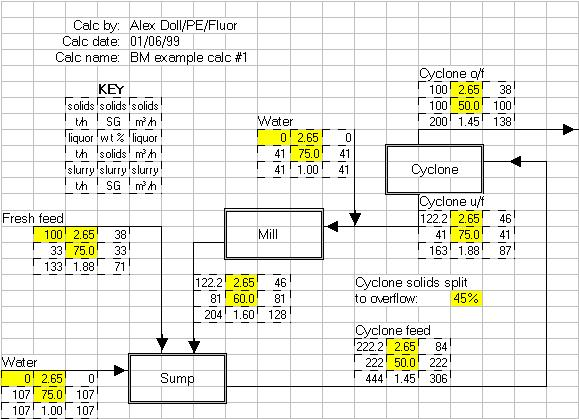Ball Mill Example #2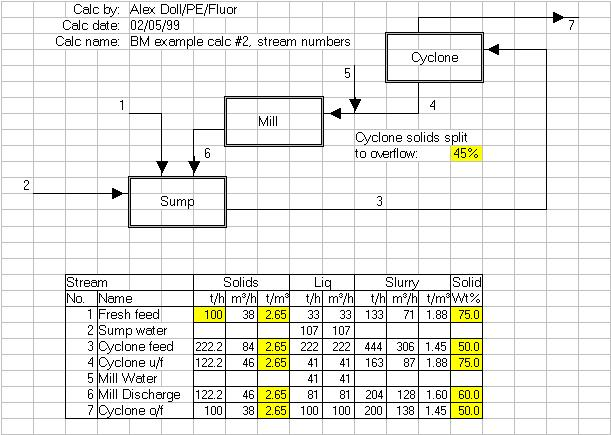### Iteration & Chaining

Number crunching is what spreadsheet based calcs do. It is therefore surprising that very few engineers who use spreadsheets to perform calculations actually understand the underlying concepts involved in a spreadsheet performing the mathematics. Metallurgical and process calculations are among the most difficult types of calculations that spreadsheets can perform, and they can sometimes highlight the strengths of the different commercial packages.

The concepts of iteration and chaining signify the order of calculation of the cells of a spreadsheet. The order of calculation is only a major concern if the spreadsheet contains a calculation with circular references (eg. circulating loads around a ball mill circuit). A spreadsheet program that examines the calculation by backward chaining to determine an optimal order of calculation (eg. Lotus 1-2-3, Corel Quattro Pro) can converge a calculation in far faster than a program that does not (eg. Microsoft Excel, various Works packages, StarOffice). In certain very complicated calculations, only the programs that use backward chaining will be able to correctly resolve the calculation regardless of the number of iterations. The non-chaining spreadsheets can get caught in a circular reference where a step change proceeds around the circular reference from cell to cell without dampening out. An example would be a closed circuit ball mill feed calculation cell that contains the value 100 on the first iteration, 0 on the second and third iterations, then back to 100 again and repeating the cycle.

### Backward Chaining

Spreadsheet programs that calculate by means of backward chaining determine the order of calculation by examining the relationships between cells. For example, if cell A1 contained the formula +B5+D7, a backward chaining spreadsheet would evaluate cells B5 and D7 before it evaluated A1. However, before it calculated B5 and D7, it would check the formulas in these cells to see if they depended on any other cells, in which case those would be evaluated first.

A spreadsheet that does not backward chain would ignore the relationships between cells. It would calculate cell A1 first using whatever values happen to be present in cells B5 and D7, even if those values are "stale". Then when B5 gets calculated, if its value changes, cell A1 will not automatically update unless the spreadsheet performs a second iteration of the calculation.

 Backward Chaining Order of Calculation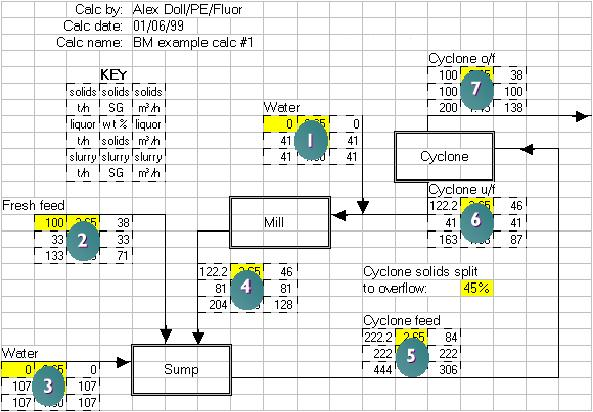Not Chained Order of Calculation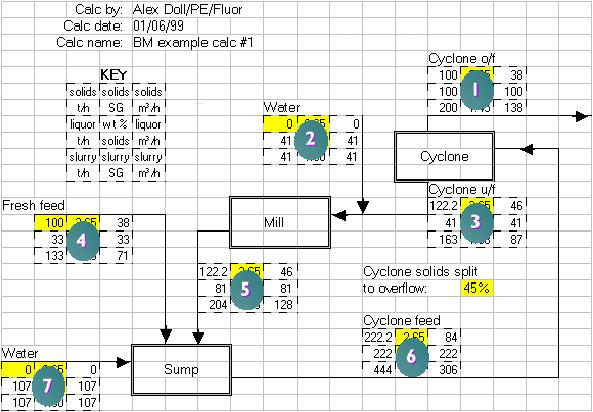#### Iteration and Convergence

Iteration is the process of repeating the evaluation of a spreadsheet until it "converges".

Convergence is defined as when two consecutive iterations of a spreadsheet yield results that are approximately the same. Some programs (eg. Microsoft Excel) allow the user to define convergence as a relative difference of, for example, less that 0.1% in all values relative to the previous iteration. Other programs (eg. Lotus 1-2-3) lack this ability to define a convergence criteria, in which case the user must define a set number of iterations for the computer to process and then examine the results visually to determine if the calculation has converged.

Calculations that involve circulating loads (like the ball mill example in an earlier section) must be constructed correctly in order for them to converge. Calculations that contain flaws in logic, imbalances around unit operations, "forgotten" streams, and other errors may find that the differences in values between successive iterations may increase rather than decrease. This yields a calculation whose values are non-converging (tending towards infinity). It is important that the engineer specify an absolute maximum number of iterations that a spreadsheet performs on a calculation so that if the calculation is non-converging, the engineer may save the calculation before infinite values begin to appear in cells.

Non-converging spreadsheets are usually the result of errors in some cells within the calculation or a faulty understanding of the system being modelled in the spreadsheet. In some very rare instances, non-convergence may also be due to the inability of non-backward chaining spreadsheets to correctly calculate circular references.

Some of the quality assurance tricks outlined later in this paper, especially double-entry accounting and alarms, can greatly assist the engineer in creating calculations that converge reliably.

#### Balancing

Balancing of calculations is concept almost unique to process engineering. Unlike convergence, a calculation is said to balance when, for all commodities, the sum of all the inputs to every unit operation is equal to the sum of all the outputs and reactions for the same unit operation. In simpler language, a calculation balances when what whatever you put into something comes out again or reacts.

Balanced spreadsheets are almost always converged, but are not necessarily correct. If the calculation is set up wrong (incorrectly mimics the process being modelled), then the results of a balanced and converged calculation will not correctly mimic reality.

The following example demonstrates two iterations of two similar calculations. The first is balanced (the sum of the inputs equals the sum of the outputs) but is not converged (the values of the internal slag recycle are changing significantly between the two iterations). The second is converged (the values do not change significantly between the two iterations) but is not balanced (there is an error in the calculation or there is another output stream that has not been accounted for).

 Example of Balance Without Convergence Example of Convergence Without Balance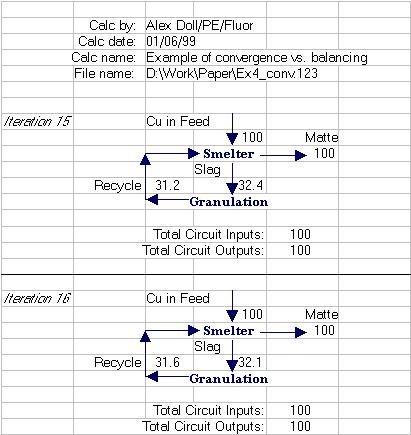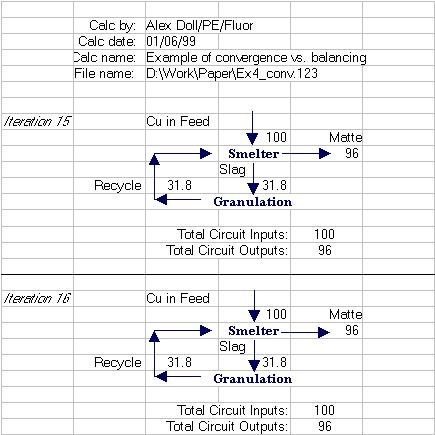### Double-entry Accounting

There are two key concepts to double entry accounting: any critical value in a calculation should be calculated in two independent ways (and the two answers compared), and, in the case a material balance, the sum of the inputs should equal the sum of the outputs and reactions for any given unit operation and for the entire plant (the calculation must balance).

Mass and energy balance calculations are easy to check as long as the calculation is set up with discrete unit operation inputs and outputs. Including a "sum of inputs" and "sum of outputs and reactions" check around all unit operations will provide a big check on whether or not there are any problems in a given spot. Similarly, a "sum of inputs" and "sum of outputs and reactions" table should be developed for the entire plant being modelled.

A "sum of inputs" check involves either a table or a SUM statement that adds all the inputs of a particular commodity into a unit operation. Each significant commodity (eg. solids, liquor, copper, sulphate) should have its own SUM statement. The corresponding "sum of outputs and reactions" is created the way, except that reactions that create or destroy the commodity must be accounted for. Reactions that destroy the commodity will be expressed as being positive, whilst reactions that create the commodity will be expressed as being negative.

The engineer must carefully choose the commodity being checked to ensure that the values entering and exiting a unit operations are actually comparable. Avoid the use of volumetric flows for commodities as small fluctuations in temperature or pressure can affect the flows entering or leaving a unit operation. Mass flows are the best choice as they are not subject to fluctuations with ambient conditions.

 Ball Mill Circuit with Double Entry Accounting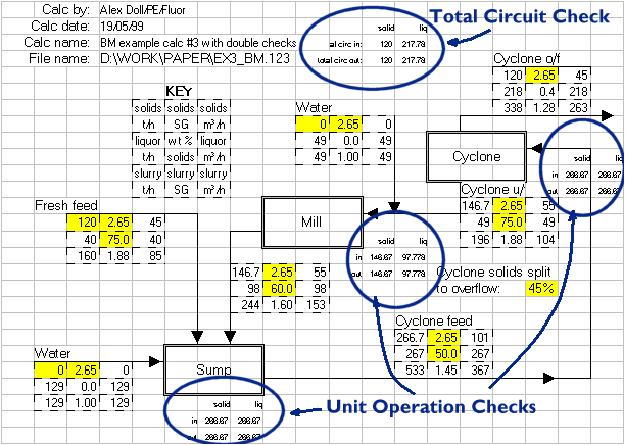The ball mill example #3 contains input and output sum tables at each major unit operation. In this example, the two commodities that are being checked are tonnes/hour of solids and tonnes/hour of liquor.

 Heat Balance Example with Double Entry Accounting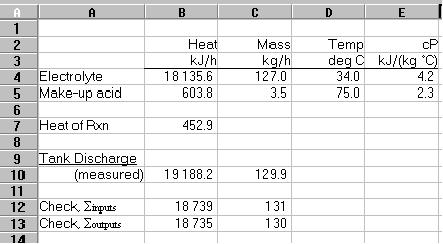A second example, consider the heat balance around a mixing vessel. Suppose the two heat inputs (cells B4 and B5) enter a vessel and undergo an exothermic reaction. The heat in the single output stream (cell B10) is calculated by a temperature measurement. The heat of reaction is calculated by a complicated fifth order differential equation (in cell B7). In this example, the "sum of inputs" would be @SUM(B4,B5) and the sum of outputs would be @SUM(B10,-B7). Note that because the reaction creates the commodity (aka heat), it is expressed as a negative number in the output sum statement.

In this example, one would expect to see a small difference between the two sums. This may be considered as unaccounted heat flows (eg. heat flow through the vessel wall), as experimental error (the temperature measurement was not accurate), or as the normal difference between theory and reality (the fifth order equation not perfectly matching reality). By displaying the two sums side-by-side, the engineer can visually gauge the difference in the inputs and outputs and decide whether or not the values are close enough to be acceptable.

### Modelling of Unit Operations

Flowsheets are useful for giving an overall view of what is happening in a calculation, but they do not afford a lot of space for doing complicated calculations. Rather than clutter the flowsheet, it is recommended that each major unit operation be carefully drawn out on its own page within the spreadsheet. The inputs to this unit operation page should be drawn from the flowsheet (or from the unit operation pages of the equipment feeding this unit operation) and the flowsheet should show the outputs from this unit operation.

Put a small sketch or flowchart of what is happening within a unit operation page. Just as a flowsheet or diagram aids in understanding the overall calculation, diagrams on the unit operation pages assist in understanding the nature of the inputs, outputs and calculation methods of this unit operation. Large calculations involving a large number of unit operations will require careful management of the inputs and outputs between units to ensure that the flow of the calculation matches the piping network in the process plant. Use of double-entry accounting can be critical in identifying when a pipeline in the plant has been "missed" in the calculation.

## Alarms

Automation of the debugging of calculations often provides better spreadsheets, faster. One of the key tools in automation of debugging is placing alarms in the calculation that are triggered when certain key cells have values outside of an acceptable range. Alarms can also be used to compare values and report nonsense conditions like pipes with different feed and discharge rates.

### Laying Alarms

Alarms are simple IF statements that test for bad conditions and display messages if they are present. A typical alarm will look like this:

@IF(H21>100,"Tank Overflowing!","")

The condition being tested is the value in cell H21. If it is greater than 100, then the cell will display the message "Tank Overflowing!", otherwise it will display nothing. This is the key concept in an alarm, it will stay blank until it is triggered.

Alarms are best used to highlight conditions in the calculation that may appear to be valid, but that simply won't work in the real world. Examples of conditions that alarms can be used to announce are negative tank levels, overflowing tanks, backward flow down a pipe, and discrepancies in double entry accounting.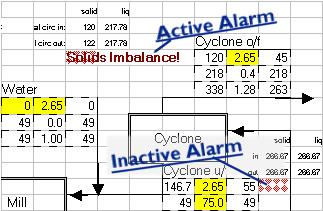It is often necessary to denote the presence of an alarm, even if it is inactive. This will avoid the alarm's cell being mistaken for being blank and having the contents overwritten. Changing the shading of the cell to a light orange colour is recommended.

Alarms are commonly used to compare values; for example, comparing the sum of inputs to a unit operation to the sum of outputs for a unit operation. There is a trick to performing comparisons: what must be tested is not the equality of the two values, but rather their relative difference. Consider the example of the sum of inputs (cell D5) equals 8.00 and the sum of outputs (cell D6) equals 8.0001. An alarm written as @IF(D5=D6,"Error","") will almost never shut off as there will always be minor differences in cell values when a calculation has converged. This alarms is better written as @IF(@ABS(D5-D6)<0.01,"Error","") where the 0.01 is the engineer's arbitrary choice of what constitutes a "significant" difference.

The person running the spreadsheet must be made aware that something bad has happened when an alarm has been triggered. This is best done by changing the text formatting of the alarm cell so that the alarm appears in a large, high-contrast red, bold print. Alarms, if they are triggered, should be located on the spreadsheet in positions where they are highly visible and where they will appear on the print out. This will alert people viewing the results of the spreadsheet that something unusual is happening in the calculation.

### Combining Alarms

Large calculations may encompass large areas of a spreadsheet and be several pages deep. It is important to keep the alarm cells close to the part of the calculation that generates the values being monitored in the alarm. This means that the alarms may be buried several pages deep on complicated calculation and may not be visible when they are triggered.

It is a good practice to have a single status area where the progress of the whole calculation may be monitored. Alarms can be "concatenated" (aka 'added') together to give an overall alarm status for a given page. A formula to concatenate alarms operates the same way as a formula to add numbers, except that the "&" symbol is used rather than the "+" symbol. For example, if there were alarms in cells E4, I8 and O10 of a page, the overall alarm cell for the page would look like:

+E4&I8&O10

Note the beginning "+" sign to tell the spreadsheet that this is a formula. This example is formatted for Lotus 1-2-3; the same formula for Microsoft Excel would use "=" to tell the spreadsheet that this is a formula. So, in Excel the example would look like:

=E4&I8&O10

Even placing these overall page status alarms may not be visible enough. If the calculation includes several pages, then it may be necessary to built a single all-encompassing alarm cell located in a highly visible part of the spreadsheet (for example, on the main page of the spreadsheet). The form of this alarm is similar to the formulas above, except that it should be the overall alarms for the various pages that get concatenated. For example, if each page has its overall alarm cell in the A1 cell, then the all-encompassing alarm cell would look like this:

+A:A1&B:A1&C:A1&D:A1

## Error Trapping

Error values in spreadsheets are created by such operations a dividing by zero, taking a logarithm of a negative number, and so on. Calculations that involve a large number of circular references run a risk of becoming populated with error messages (eg. ERR or NA). Engineers should always expect that these errors will eventually creep into their spreadsheets, and therefore should take measures to protect their calculation.

### Critical Junctions

Just as a highway has intersection where bad things may happen, spreadsheets with circular calculations have junctions. A junction is defined as a cell within a spreadsheet whose formula depends on one or more downstream cells. By placing special error traps at these junction cells, the engineer may prevent errors propagating throughout a calculation.

The code for an error trap consists of an IF statement that checks for an error downstream, a default value to display if a downstream error is present, and a formula to execute if there are no errors downstream. In 1-2-3, the code will look like this:

@IF(@ISERR(H21),100,H21/100*A3)

The expression @ISERR(H21) will return "true" if the value of cell H21 is ERR. This "true" value will result in the formula returning the value '100'. If there is no error present, the @ISERR(H21) will return false, which will result the expression H21/100+A3 being evaluated instead. Note that in this example, the cell A3 is not being checked for errors. If the layout of the calculation is such that A3 is "upstream" of the cell we are calculating, then we may ignore it. If, however, the value in A3 did have a potential to cause errors to propagate to this cell, then the engineer should modify the checking routine to look for an error in cell A3 too. Changing the formula to:

@IF(@ISERR(H21+A3),100,H21/100*A3)

is sufficient as if either of H21 or A3 contain an ERR value, then the addition will result in ERR as well. Note that the spreadsheet can evaluate addition faster than multiplication, thus your spreadsheet will run faster if the formula @ISERR(H21+A3) is used instead of @ISERR(H21/100*A3).

The locations of critical junctions is crucial to the success of your error trapping. You must have enough traps at critical parts of the spreadsheet to prevent errors propagating, but not so many traps that they begin to slow down the calculation of the spreadsheet. In the ball mill circuit example used previously, the cyclone underflow and sump discharge cells are the critical junctions and should be fitted with error traps. All the other cells can operate without error traps as any errors that they generate would have to travel through the two critical junctions that already have traps.

Choose default values carefully. They should sized approximately to what the formula would normally result in (if values are too large or small, then other downstream formulas may end up evaluating to ERR). The value should also be readily visible so that you have a visual clue that something is wrong and that the trap has been sprung (for example, a cell with the value 100.00 will look suspicious whereas 93.70 would appear "normal"). If the trap is sprung, then the double-entry accounting and alarms discussed in earlier chapters should alert you to the fact that something is wrong (it is important that they be built into the calculation).

## Error Recovery

It is possible to clean up a spreadsheet once it has become infested with errors; but it is a lot of work.

The first step in recovering the calculation is to install error traps at critical junctions as described in the previous section. This will help isolate the part of the calculation that is generating the error and will hopefully allow the other parts of the spreadsheet to operate as normal. Keep isolating smaller and smaller parts of the calculation until a small part of the calculation is now free of errors. Continue adding error traps until larger sections of the spreadsheet begin to operate normally and you can identify the part of the calculation that is generating the error. Then fix the formula that is generating the error and hopefully the calculation will return to normal.

A shortcut that can be used with recovering from propagated errors is to hard-wire some of the formulas. Copy the formula to a nearby blank cell and then overwrite the original formula with a value similar to what it will normally evaluate to. This will trick the downstream formulas into calculating relatively normal values while the engineer is hunting down the rest of the errors. Once the spreadsheet has been cleaned of errors, then the copied formulas should be re-copied over the manually entered values in the calculation.

## Iteration Dampening

Iteration dampening is a technique of forcing a complicated calculation to converge quicker than it would do on its own. This technique is dangerous, though, and must be used in conjunction with the double entry accounting and alarms that have been described previously.

There are often key cells in a calculation that have values that vary widely as a calculation iterates towards convergence. These cells can be forced to not vary outside of a given range; for example, a cell that should yield a small, positive number can be limited so that its value does not drop below zero. This is done using an IF statement:

@IF((A3+H21/100)<0,0,A3+H21/100)

Note that this expression does not place an upper limit on the value in the cell. It is usually not necessary to put an upper limit on a cell's value as the lower limit is usually enough. To make matters worse, it is common that the units of measure used in an engineering calc will change as the calculation evolves, and a change in units usually causes more grief with an upper limit than with a lower limit.

The calculation will not balance when iteration dampening is operating in a cell. It is important to have double entry accounting and alarms set up around any cell with iteration dampening code to alert the user when dampening is in progress. It is possible for iteration dampening to allow a calculation to converge without balancing so the engineer must write the spreadsheet in a way that detects and announces the problem.

## Conclusion

Computer spreadsheets are powerful tools that can be extremely useful to engineers. They may also be extremely dangerous if calculations are not properly implemented. The order of calculation of a spreadsheet program is sometimes critical to solving calculations using circular references,

Simple tools like layout based calculations and alarms can make the communication of ideas in a calculation more successful. Other simple tools, like double entry accounting and error trapping, can make the results of a calculation less prone to errors.